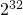# Integers in Perl 6

The language Perl 6 has been announced to be production ready by the beginning of this year. Its implementation is Rakudo, while the Perl 6 programming language itself is an abstract language definition that allows any language implementation that passes the test suite to call itself an Perl 6 implementation. The idea is not totally new, we see the Ruby language being implemented more than once (Ruby, JRuby, Rubinius, IronRuby), but we can also learn from the Ruby guys that it is a challenge to keep this up to date and eventually it is likely that one implementation will fall back or go its own way at some point of time.

Perl 6 is also called „Perl“ as part of its name, but quite different from its sister language Perl, which is sometimes called „Perl 5“ to emphasize the distinction, so it is absolutely necessary to call it „Perl 6“ or maybe „Rakudo“, but not just „Perl“.

Even though many things can be written in a similar way, a major change to Perl 5 is the way of dealing with numeric types. You can find an article describing Numeric Types in Perl . So now we will see how to do the same things in Perl 6.

Dealing with numeric types in Perl 6 is neither like in Perl 5 nor like what we are used to in many other languages.

So when we just use numbers in a naïve way, we get long integers automatically:

```my \$f = 2_000_000_000;
my \$p = 1;
loop (my Int \$i = 0; \$i < 10; \$i++) {
say(\$i, " ", \$p);
\$p *= \$f;
}
```

creates this output:

```0 1
1 2000000000
2 4000000000000000000
3 8000000000000000000000000000
4 16000000000000000000000000000000000000
5 32000000000000000000000000000000000000000000000
6 64000000000000000000000000000000000000000000000000000000
7 128000000000000000000000000000000000000000000000000000000000000000
8 256000000000000000000000000000000000000000000000000000000000000000000000000
9 512000000000000000000000000000000000000000000000000000000000000000000000000000000000
```

This is an nice default, similar to what Ruby, Clojure and many other Lisps use, but most languages have a made a choice that is weird for application development.

Now we can also statically type this:

```my Int \$f = 2_000_000_000;
my Int \$p = 1;
loop (my Int \$i = 0; \$i < 10; \$i++) {
say(\$i, " ", \$p);
\$p *= \$f;
}
```

and we get the exact same result:

```0 1
1 2000000000
2 4000000000000000000
3 8000000000000000000000000000
4 16000000000000000000000000000000000000
5 32000000000000000000000000000000000000000000000
6 64000000000000000000000000000000000000000000000000000000
7 128000000000000000000000000000000000000000000000000000000000000000
8 256000000000000000000000000000000000000000000000000000000000000000000000000
9 512000000000000000000000000000000000000000000000000000000000000000000000000000000000
```

Now we can actually use low-level machine integers which do an arithmetic modulo powers of 2, usuallyor:

```my int \$f = 2_000_000_000;
my int \$p = 1;
loop (my Int \$i = 0; \$i < 10; \$i++) {
say(\$i, " ", \$p);
\$p *= \$f;
}
```

and we get the same kind of results that we would get in java or C with (signed) long, if we are on a typical 64-bit environment:

```0 1
1 2000000000
2 4000000000000000000
3 -106958398427234304
4 3799332742966018048
5 7229403301836488704
6 -8070450532247928832
7 0
8 0
9 0
```

We can try it in Java. I was lazy and changed as little as possible and the "\$" is allowed as part of the variable name by the language, but of course not by the coding standards:

```public class JavaInt {
public static void main(String[] args) {
long \$f = 2_000_000_000;
long \$p = 1;
for (int \$i = 0; \$i < 10; \$i++) {
System.out.println(\$i + " " +  \$p);
\$p *= \$f;
}
}
}
```

We get this output:

```0 1
1 2000000000
2 4000000000000000000
3 -106958398427234304
4 3799332742966018048
5 7229403301836488704
6 -8070450532247928832
7 0
8 0
9 0
```

And we see, with C# we get the same result:

```using System;

public class CsInt {

public static void Main(string[] args) {
long f = 2000000000;
long p = 1;
for (int i = 0; i < 10; i++) {
Console.WriteLine(i + " " +  p);
p *= f;
}
}
}
```

gives us:

```0 1
1 2000000000
2 4000000000000000000
3 -106958398427234304
4 3799332742966018048
5 7229403301836488704
6 -8070450532247928832
7 0
8 0
9 0
```

If you like, you can try the same in C using signed long long (or whatever is 64 bits), and you will get the exact same result.

Now we can simulate this in Perl 6 also using Int, to understand what int is really doing to us. The idea has already been shown with Ruby before:

```my Int \$MODULUS = 0x10000000000000000;
my Int \$LIMIT   =  0x8000000000000000;
sub mul(\$x, \$y) {
my Int \$result = (\$x * \$y) % \$MODULUS;
if (\$result >= \$LIMIT) {
\$result -= \$MODULUS;
} elsif (\$result < - \$LIMIT) {
\$result += \$MODULUS;
}
\$result;
}

my Int \$f = 2_000_000_000;
my Int \$p = 1;
loop (my Int \$i = 0; \$i < 10; \$i++) {
say(\$i, " ", \$p);
\$p = mul(\$p, \$f);
}
```

and we get the same again:

```0 1
1 2000000000
2 4000000000000000000
3 -106958398427234304
4 3799332742966018048
5 7229403301836488704
6 -8070450532247928832
7 0
8 0
9 0
```

The good thing is that the default has been chosen correctly as Int and that Int allows easily to do integer arithmetic with arbitrary precision.

Now the question is, how we actually get floating point numbers. This will be covered in another blog posting, because it is a longer story of its own interest.